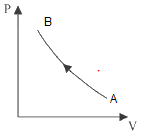# P-V Diagram

## P-V Indicator Diagram

• Only two thermodynamic variables are sufficient to describe a system because third variable can be calculated from equation of state of the system.
• P-V Indicator Diagram is just a graph between pressure and volume of a system undergoing an operation.
• When a system undergoes an expansion from state A (P1 V1) to a state B (P2V2) its indicator diagram is shown as follows.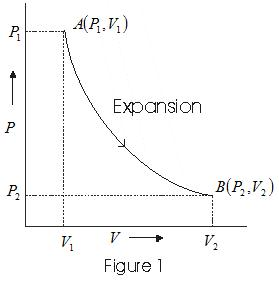• In case of compression system at state A(P1 V1) goes to a state B(P2V2) its P-V diagram is as follows.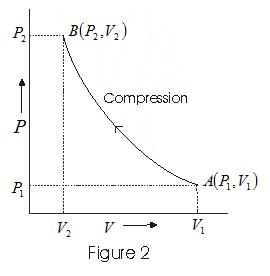• Intermediate states of system are represented by points on the curve.
• The pressure volume curve for a fixed temperature is called isotherm.

## Solved Example of PV diagram

Question 1
Consider P-V diagram for an ideal gas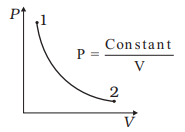Draw the corresponding T-P diagram
Solution
here
$P= \frac {constant}{V}$
or
$PV= constant$
So it means temperature is constant.So here is the TP diagram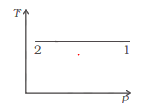Question 2
Draw the corresponding P-V diagram for the given T-V diagram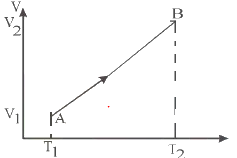Solution
Since TV diagram is a straight line
T/V =constant
it means Pressure is constant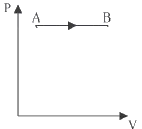Question 3
Draw the corresponding P-V diagram for the given T-V diagram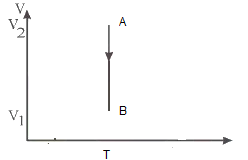Solution
Since T is constant
PV diagram should be isotherm